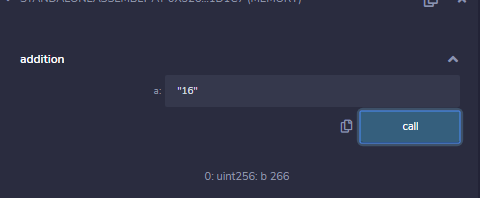# Solidity – Assembly

Assembly or Assembler language indicates a low-level programming language that can be converted to machine code by using assembler. Assembly language is tied to either physical or a virtual machine as their implementation is an instruction set, and these instructions tell the CPU to do that fundamental task like adding two numbers.

Solidity has an option to write an assembly language code inside the smart contract’s source code. With the help of Solidity assembly, we can directly interact with the EVM using the opcodes. Assembly provides more control over some logic which cannot be possible using only solidity, like pointing to the specific memory block. One of the main advantages is that it reduces the cost of the gas used to deploy the contract. Solidity has two ways to implement the assembly language:

Inline Assembly: Inline assembly code can be written inside solidity code for more fine-grain control and especially used for enhancing the language via creating new libraries. Inline assembly can be inserted in between solidity statements in a way that EVM can understand. It can also be used when the optimizer is not able to produce efficient code. Solidity becomes easier when features like assembly local variables, functions calls, switch statements, if statements, loops, etc are used.
Syntax:

```assembly{
// assembly language statements
}
```

Example: In the below example, a contract with a function is created, where the inline assembly code is located inside the function.

## Solidity

 `// Solidity program to demonstrate  ` `// Inline Assembly ` `pragma solidity ^0.4.0; ` ` `  `// Creating a contract ` `contract` `InlineAssembly { ` ` `  `    ``// Defining function  ` `    ``function add(uint a) view returns (uint b) { ` ` `  `        ``// Inline assembly code ` `        ``assembly { ` ` `  `            ``// Creating a new variable 'c' ` `            ``// Calculate the sum of 'a+16'  ` `            ``// with the 'add' opcode ` `            ``// assign the value to the 'c' ` `            ``let c := add(a, 16) ` ` `  `            ``// Use 'mstore' opcode to  ` `            ``// store 'c' in memory  ` `            ``// at memory address 0x80 ` `            ``mstore(0x80, c) ` `            ``{ ` ` `  `                ``// Creating a new variable' ` `                ``// Calculate the sum of 'sload(c)+12'  ` `                ``// means values in variable 'c'  ` `               ``// with the 'add' opcode ` `               ``// assign the value to 'd' ` `                ``let d := add(sload(c), 12) ` `  `  `                 ``// assign the value of 'd' to 'b' ` `                ``b := d ` `  `  `            ``// 'd' is deallocated now ` `            ``}  ` `     `  `            ``// Calculate the sum of 'b+c' with the 'add' opcode ` `            ``// assign the value to 'b' ` `            ``b := add(b, c) ` ` `  `        ``// 'c' is deallocated here ` `        ``}  ` `    ``} ` `} `

Output :Sometimes writing assembly code is more difficult because will not perform checks, that’s why it is better to use only when things are complex and you have an idea about what you are doing.

Standalone Assembly: Standalone assembly is planned for making an intermediate language for the solidity compiler. It was supported for recent compatibility but it is no more documented in the solidity documentation. Programs written in a standalone assembly are readable even if the code is been generated by a solidity compiler. The control flow should be easy, for optimization and formal verification.

Example: In the below example, the contract and a function are created to demonstrate the concept of Standalone assembly.

## Solidity

 `// Solidity program to demonstrate ` `// Standalone Assembly ` `pragma solidity ^0.4.0; ` ` `  `// Creating a contract ` `contract` `StandaloneAssembly { ` ` `  `  ``// Defining a function ` `  ``function add(uint a) view returns (uint b) { ` ` `  `    ``// Initializing the variable b ` `    ``b = 10; ` ` `  `    ``// Executing for loop till the condition is met ` `    ``for` `(uint n = 0; n < a; n++) ` `      ``b = b + a; ` `  ``} ` `}`

The assembly level code for the above example is :

mstore(0x40, 0x60) // this line will store the “free memory pointer”
// dispatch function
case 0xb3de649b {
let retVal := \$memAllocate(0x20)
mstore(retVal, retData)
return(retVal, 0x20)

default { revert(0, 0) }
// allocate memory
function \$memAllocate(size) -> pos {

// function of the contract
b := 10
for { let n := 0 } lt(n, a) { n := add(n, 1) } {

Output :My Personal Notes arrow_drop_upCheck out this Author's contributed articles.

If you like GeeksforGeeks and would like to contribute, you can also write an article using contribute.geeksforgeeks.org or mail your article to contribute@geeksforgeeks.org. See your article appearing on the GeeksforGeeks main page and help other Geeks.

Please Improve this article if you find anything incorrect by clicking on the "Improve Article" button below.

Article Tags :

Be the First to upvote.

Please write to us at contribute@geeksforgeeks.org to report any issue with the above content.